# Insertion in a Doubly Linked List using C

## Insertion in a Doubly Linked List using C Language:

In this article, I am going to discuss How to Perform Insertion in a Doubly Linked List using C Language with Examples. Please read our previous article, where we discussed Doubly Linked List in C Language with Examples. At the end of this article, you will understand how to insert a new node in an existing doubly linked list.

##### Insertion in a Doubly Linked List using C Language:

There are two cases for insertion in the doubly linked list.

1. Insert Before First Node.
2. Insert at any Given Index.

Let us take an example of a doubly linked list.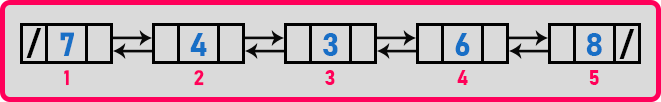This doubly linked list has 5 elements which are indexed from 1 to 5. Let us mark the position where we can insert the new nodes.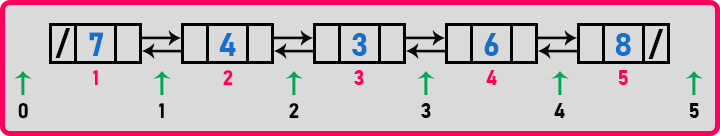Here, the green arrows and the corresponding number represent the positions where we can insert a new node. So here a total of 6 positions are possible that are indexed from o to 5 where we can insert a new node. Inserting at the 0th position is insertion before the first node. So let us see the procedure for insertion before the first node.

##### Insertion Before First Node in a Doubly Linked List:

Below are the steps required for inserting at the 0th index:

The first step is to create a new node with the help of a temporary pointer i.e. ‘t’.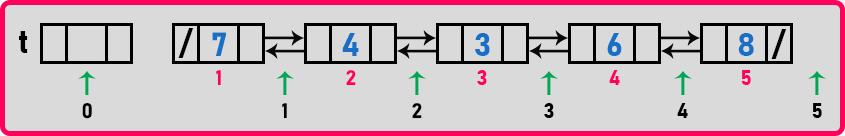Then we add some data to this node i.e. 11,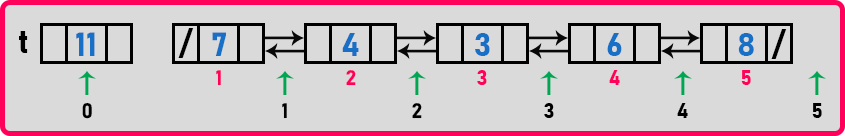Now we have to modify the links that are the prev and next pointers of the new node ‘t’. And we have to modify the prev pointer of the first node. So, in total, we have to modify 3 links.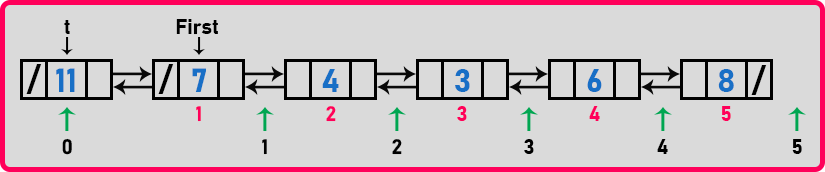Here we have modified those 3 links.

t->prev = NULL;
t->next = first;
first->prev = t;

So we have performed these 3 steps. Now we have to make the ‘t’ node the first node. So, we have to bring the first pointer to the ‘t’ node.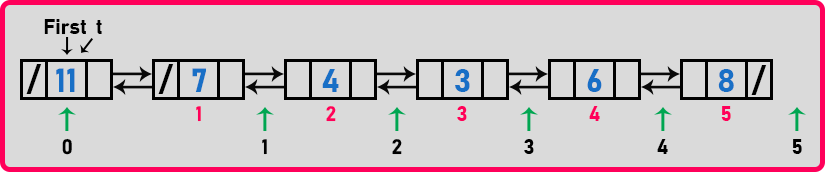Now let us write the pseudo code for this procedure.

Node *t = new Node;
t->data = x;
t->prev = NULL;
t->next = first;
first->prev = t;
first = t;

So we have performed a total of 6 steps in this procedure. 6 is constant. So the time taken for inserting a new node is constant. Now let us look at the second case.

##### Insertion at any Given Position in a Doubly Linked List: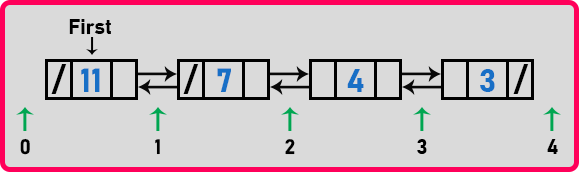Let’s say we want to insert at 2nd position. Let’s see the steps required for insertion at 2nd position. First, we have to create a new node with the help of temporary pointer ‘t’,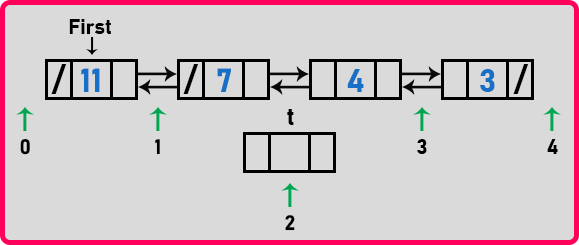Now fill the data in this new node i.e. 9,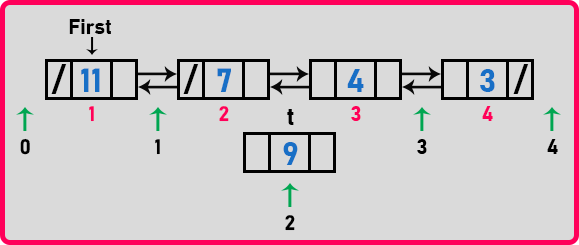This node should come between the 2nd and 3rd nodes. So what are the links we have to modify? We have to make t->next point on the 3rd node and t->prev point on the 2nd node. And 2nd node’s next should point to ‘t’ and 3rd node’s prev should point to ‘t’. So we have to modify 4 links. For modifying these nodes we should have a pointer over 2nd node. We just need one pointer, not two. Let us take a pointer ‘p’ and bring it to 2nd node.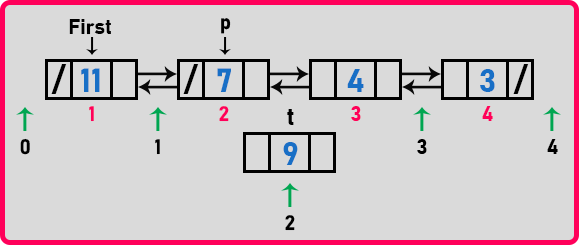Here we have just moved one time to bring the ‘p’ pointer on 2nd node. Now we have a p pointer on the 2nd node. Now we can make modifications to the links.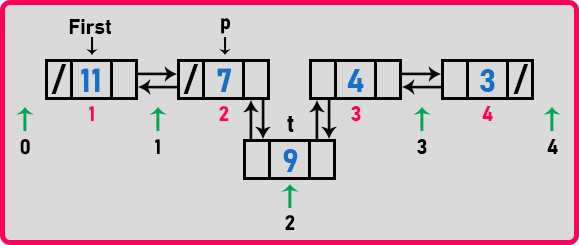t->next = p->next;
t->prev = p;
p->next->prev = t;
p->next = t;

for modifying the p->next->prev, we have to check whether there is a next node available. So we have to check for that by if-condition. We have preserved the continuation of links. We are able to move in either direction. Now let us write the pseudo code for this procedure.

Node *t = new Node;
t->data = x;
for(i = 0; i < pos-1; i++)
p = p->next;
t->next = p->next;
t->prev = p;
if(p->next)
p->next->prev = t;
p->next = t;

These are the steps for inserting a new node at a given position.

##### Analysis of Doubly Linked List Insertion:

If we are inserting before the first node, time will be constant. In the second case, time depends on the for-loop or while loop which is shifting the pointer p. If p is moving up to the last node then the time will be maximum. If you are inserting after the first node then p will move by only one step so the time will be minimum. So the minimum time is constant and the maximum time is n.

How many pointers do we require? In the case of insertion before the first, just one pointer is required otherwise 2 pointers are required for insertion at any given position.

How many links have been modified? If we are insertion before first, 3 links have been modified (if there is any first node), if the linked list is null just 2 links will be modified and if you are inserting at other positions, 4 links have been modified. Now let us write the complete program for insertion in the doubly linked list.

##### Example to Understand Insertion in Doubly Linked List using C Language:
```#include <stdio.h>
#include<stdlib.h>

struct Node {
struct Node * prev;
int data;
struct Node * next;
}* first = NULL;

void create(int A[], int n) {
struct Node * t, * last;
int i;

first = (struct Node * ) malloc(sizeof(struct Node));
first -> data = A;
first -> prev = first -> next = NULL;
last = first;

for (i = 1; i < n; i++) {
t = (struct Node * ) malloc(sizeof(struct Node));
t -> data = A[i];
t -> next = last -> next;
t -> prev = last;
last -> next = t;
last = t;
}
}

void Display(struct Node * p) {
while (p) {
printf("%d ", p -> data);
p = p -> next;
}
printf("\n");
}

int Length(struct Node * p) {
int len = 0;

while (p) {
len++;
p = p -> next;
}
return len;
}

void Insert(struct Node * p, int index, int x) {
struct Node * t;
int i;

if (index < 0 || index > Length(p))
return;
if (index == 0) {
t = (struct Node * ) malloc(sizeof(struct Node));
t -> data = x;
t -> prev = NULL;
t -> next = first;
first -> prev = t;
first = t;
} else {
for (i = 0; i < index - 1; i++)
p = p -> next;
t = (struct Node * ) malloc(sizeof(struct Node));
t -> data = x;

t -> prev = p;
t -> next = p -> next;
if (p -> next) p -> next -> prev = t;
p -> next = t;

}
}

int main() {
int A[] = {7, 4, 3, 6, 8};
create(A, 5);

Display(first);

Insert(first, 0, 11);
Insert(first, 2, 9);

printf("List After insertion: \n");
Display(first);
return 0;
}
```
###### Output: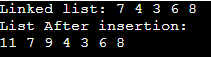In the next article, I am going to discuss Insertion in a Doubly Linked List with Examples. Here, in this article, I try to explain How to Perform Insertion in a Doubly Linked List using C Language with Examples. I hope you enjoy this Insertion in a Doubly Linked with Algorithm and Examples article.